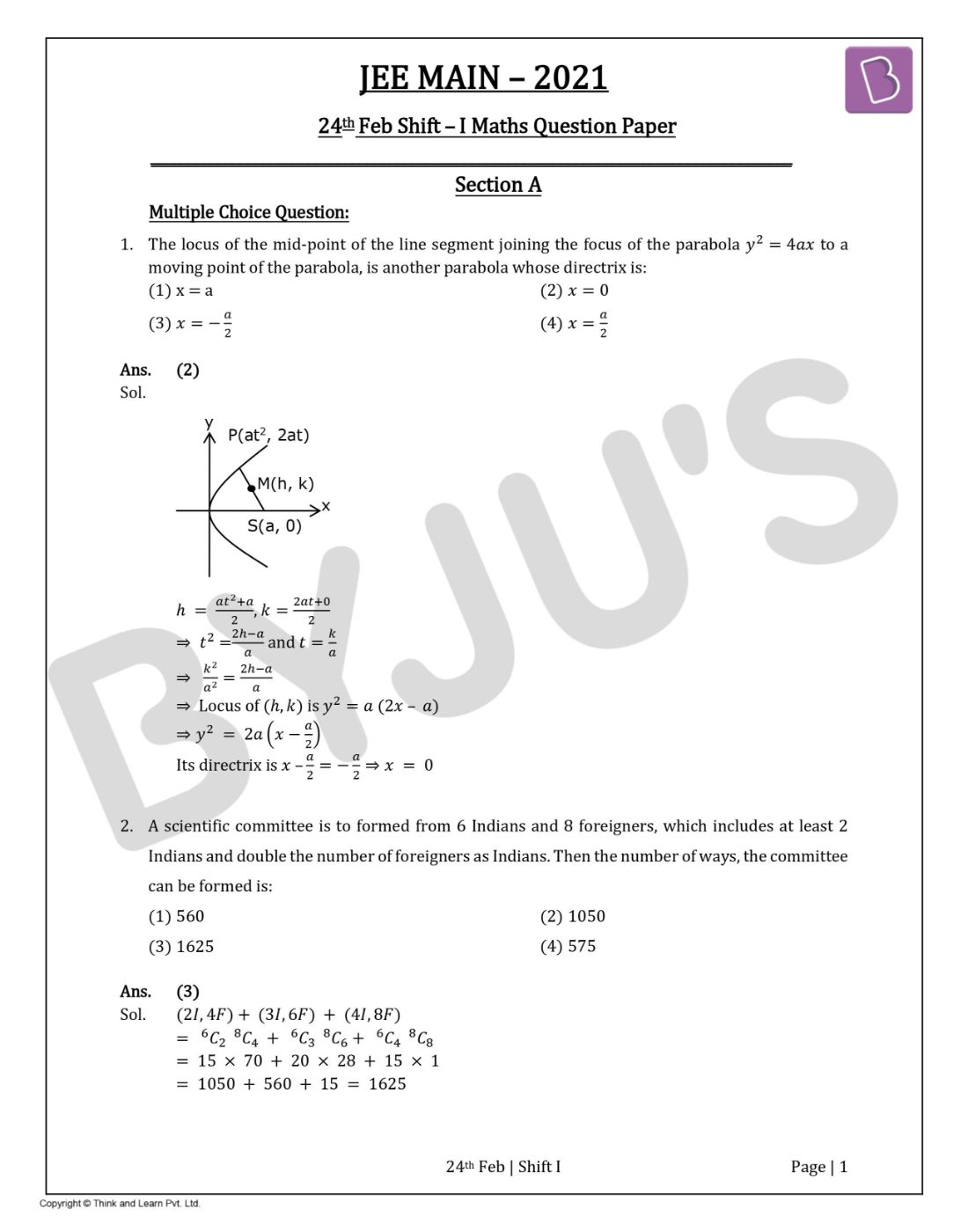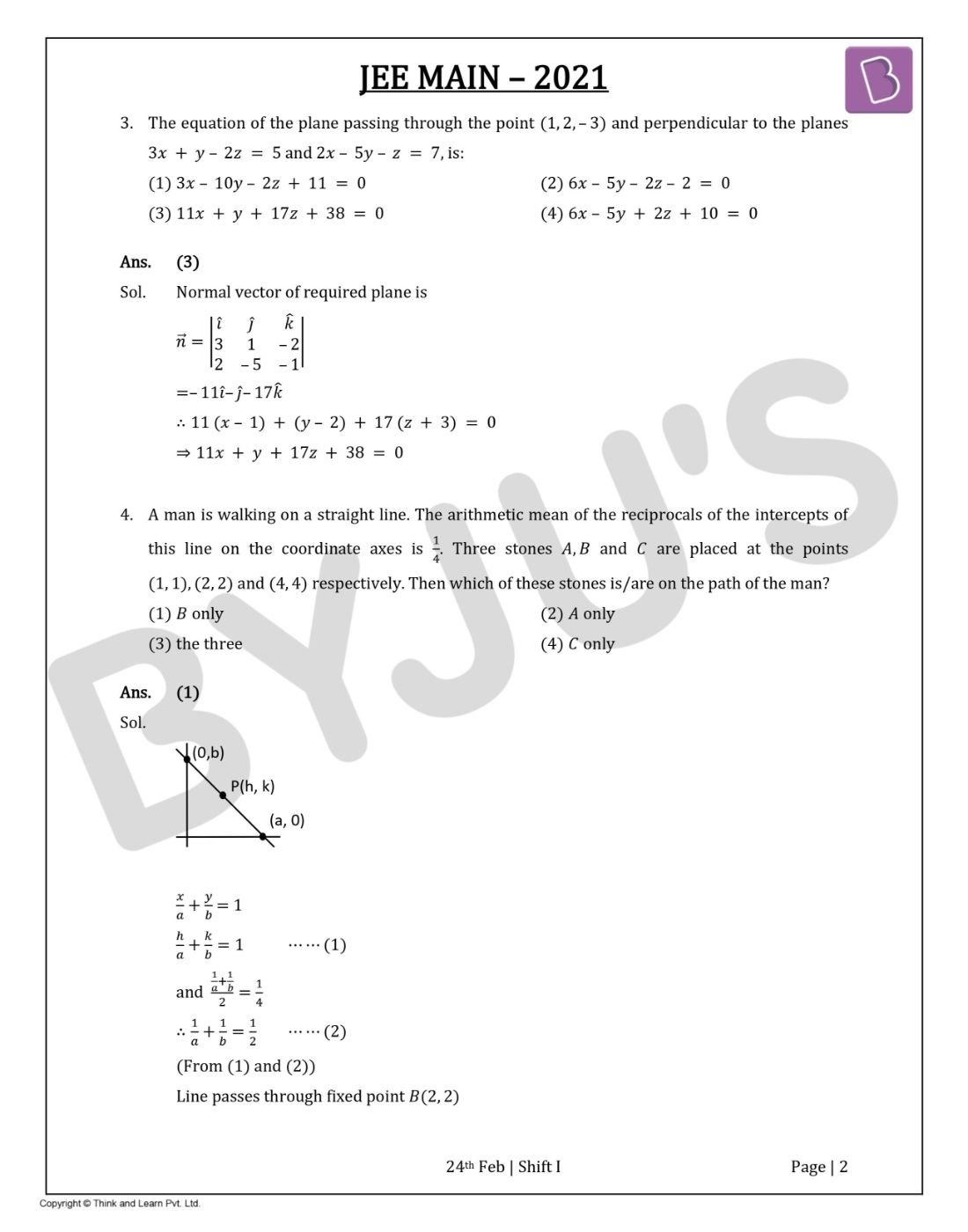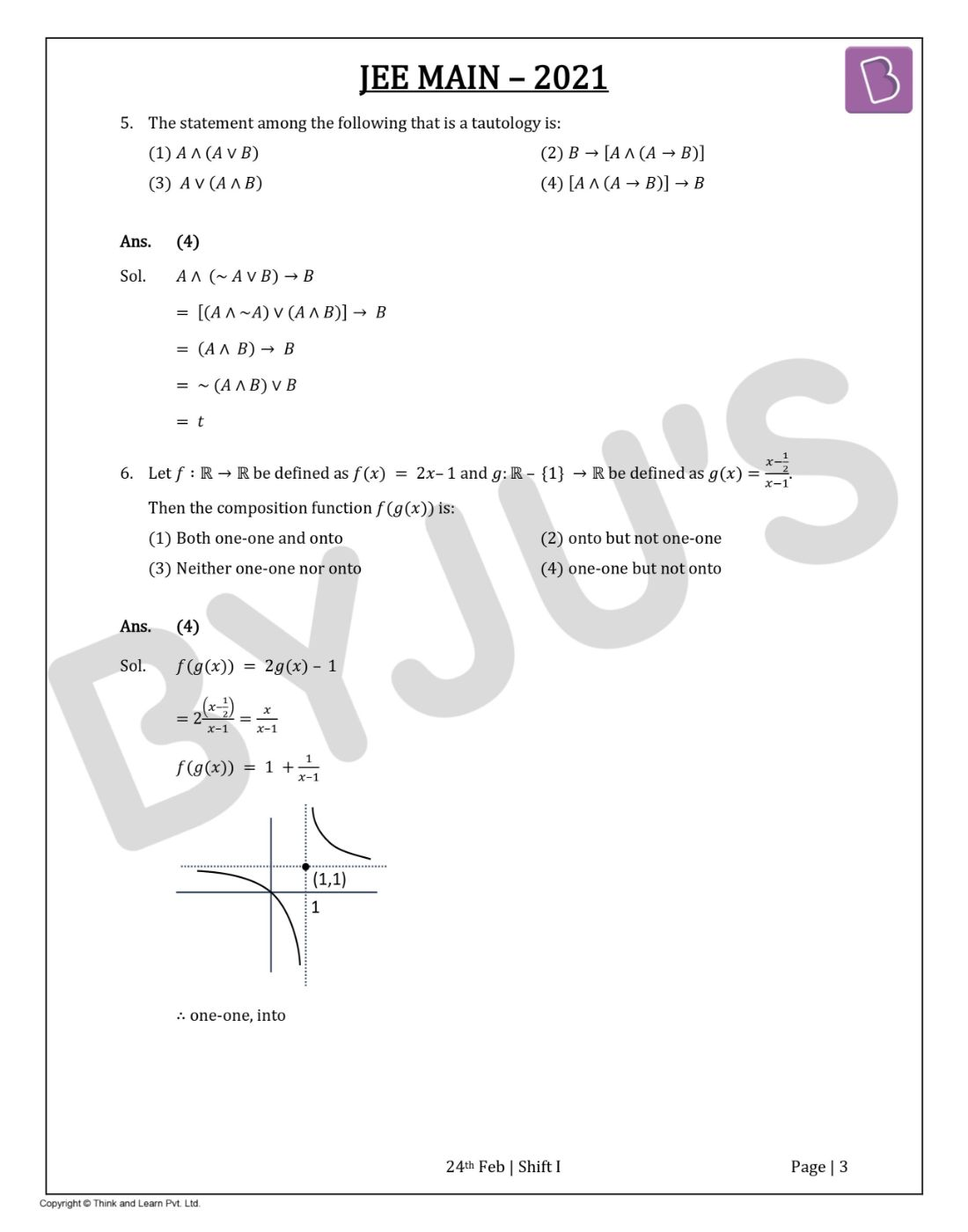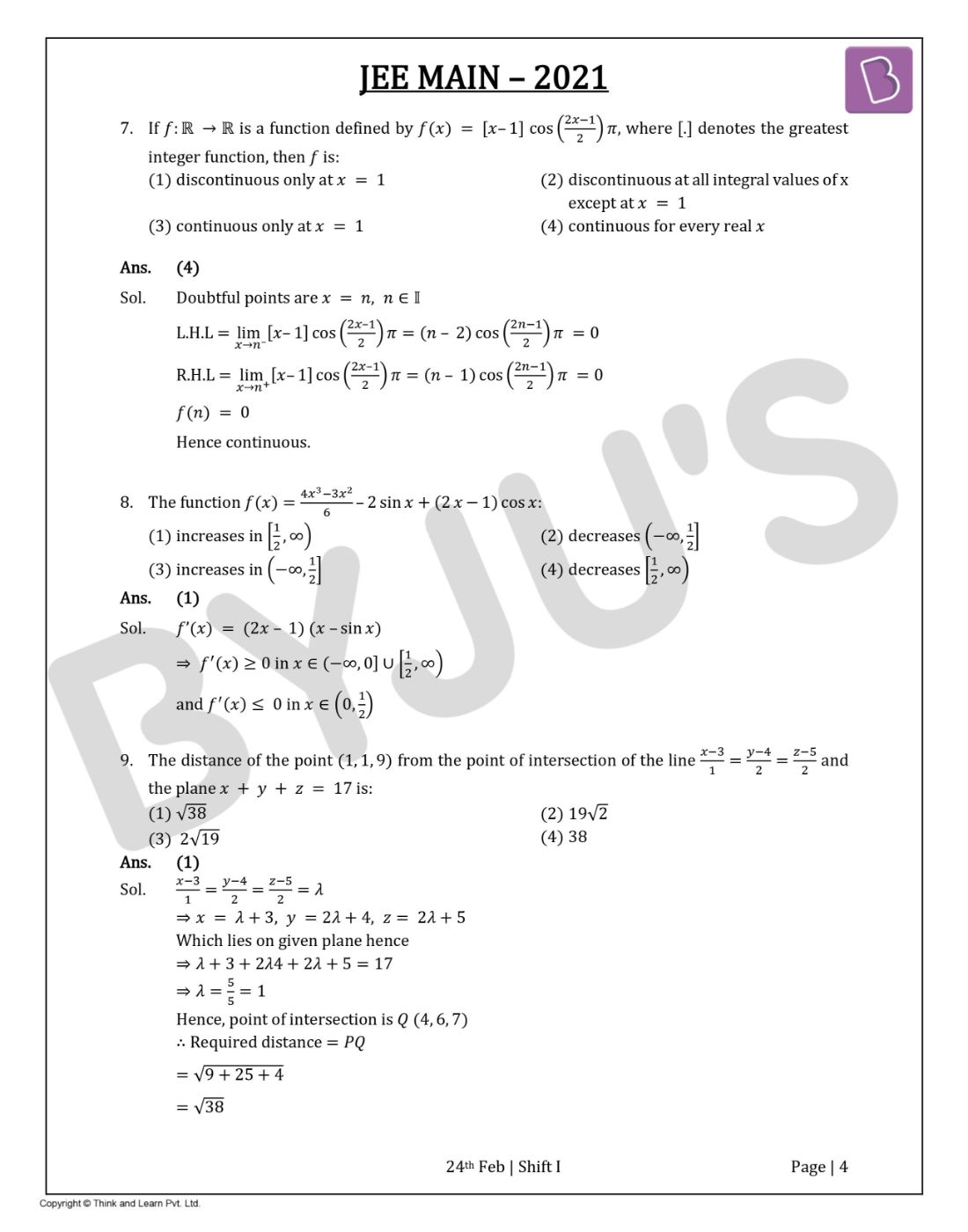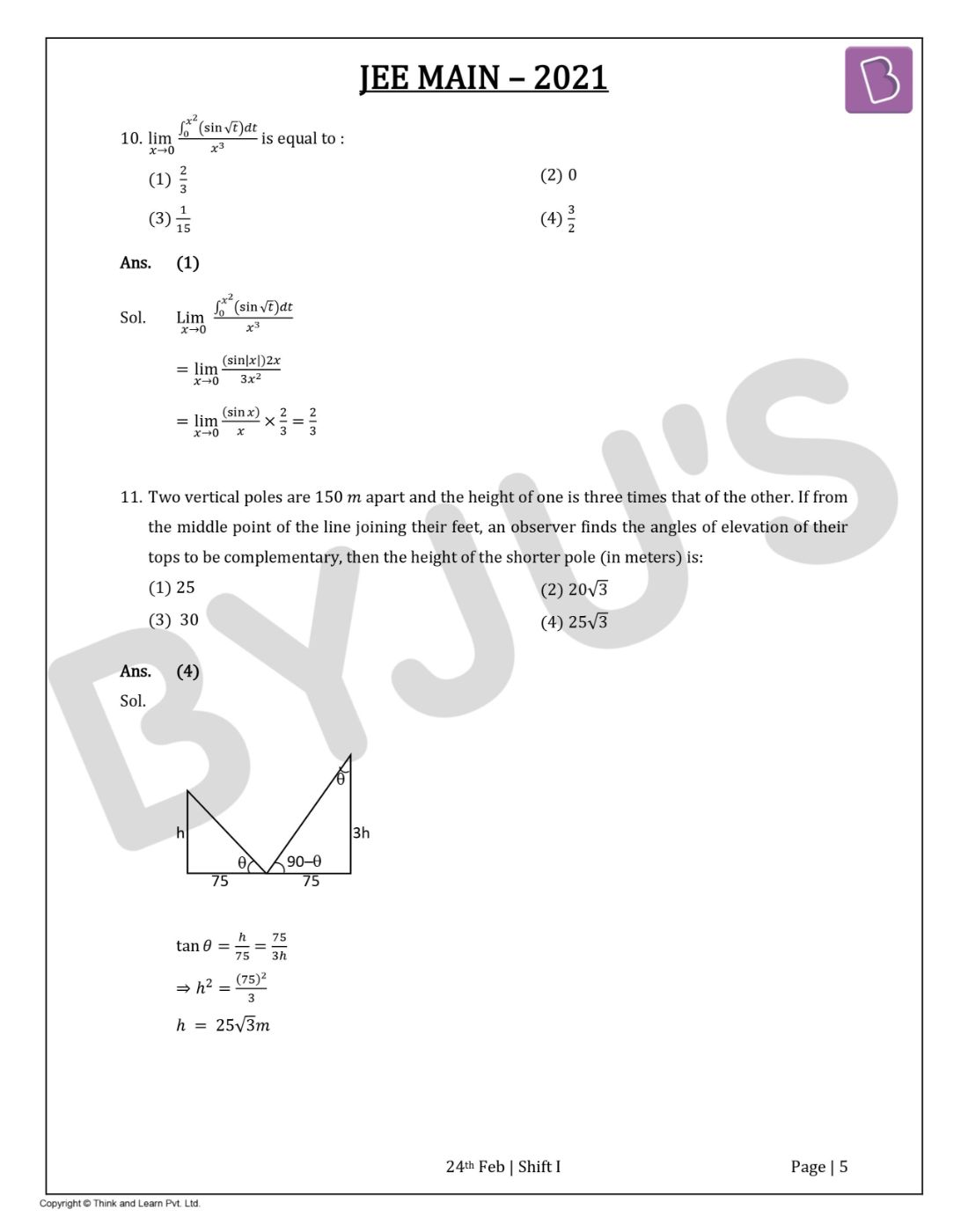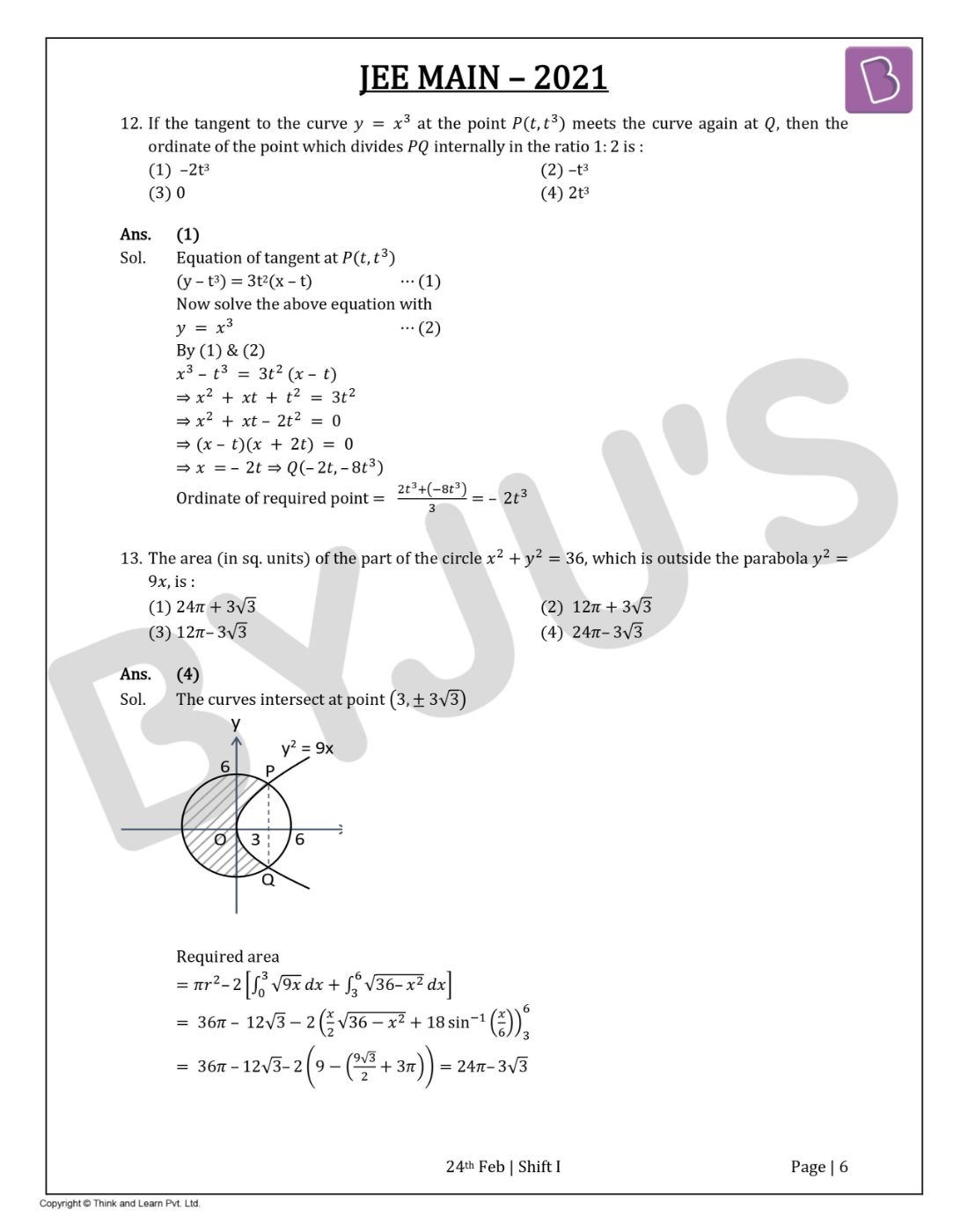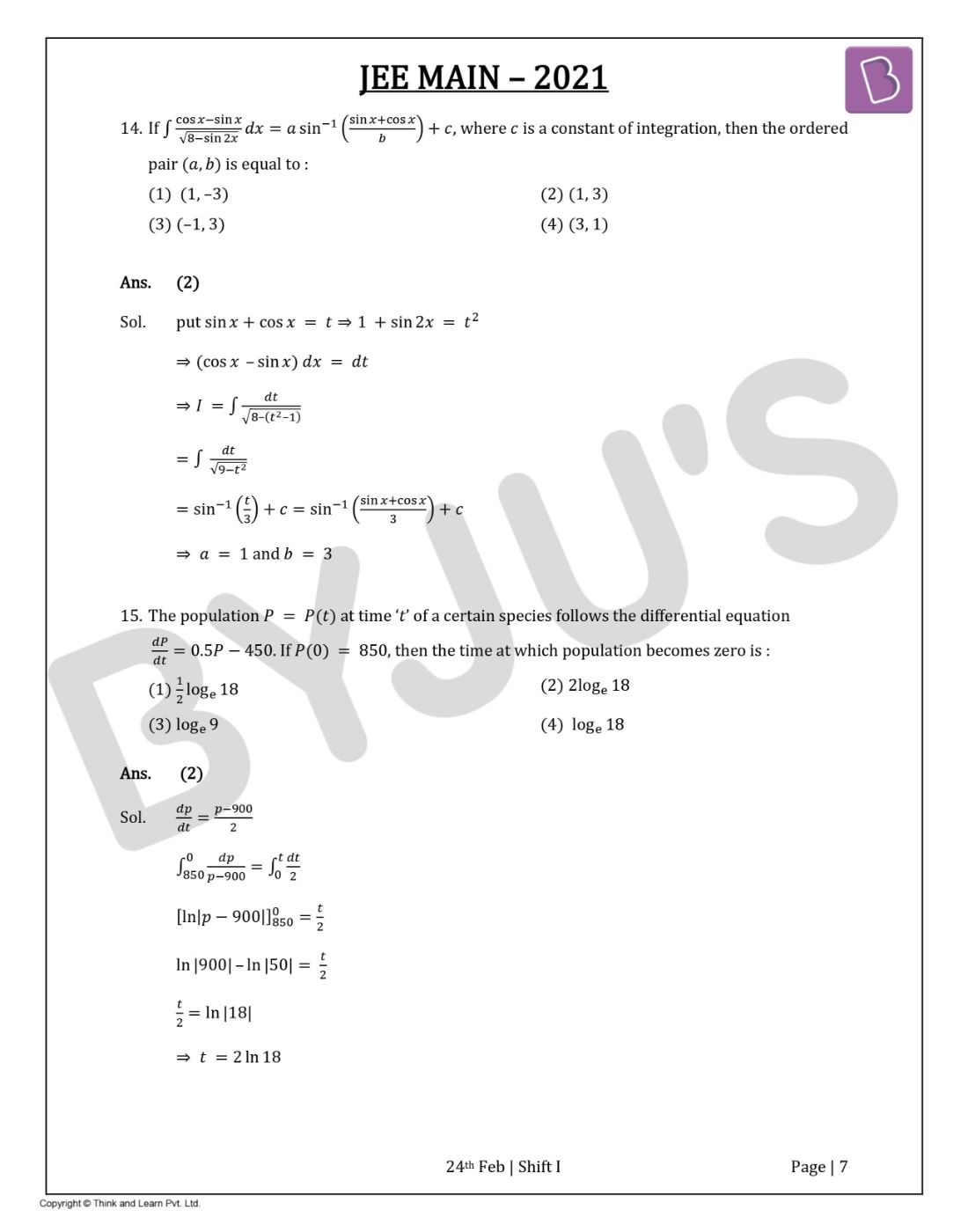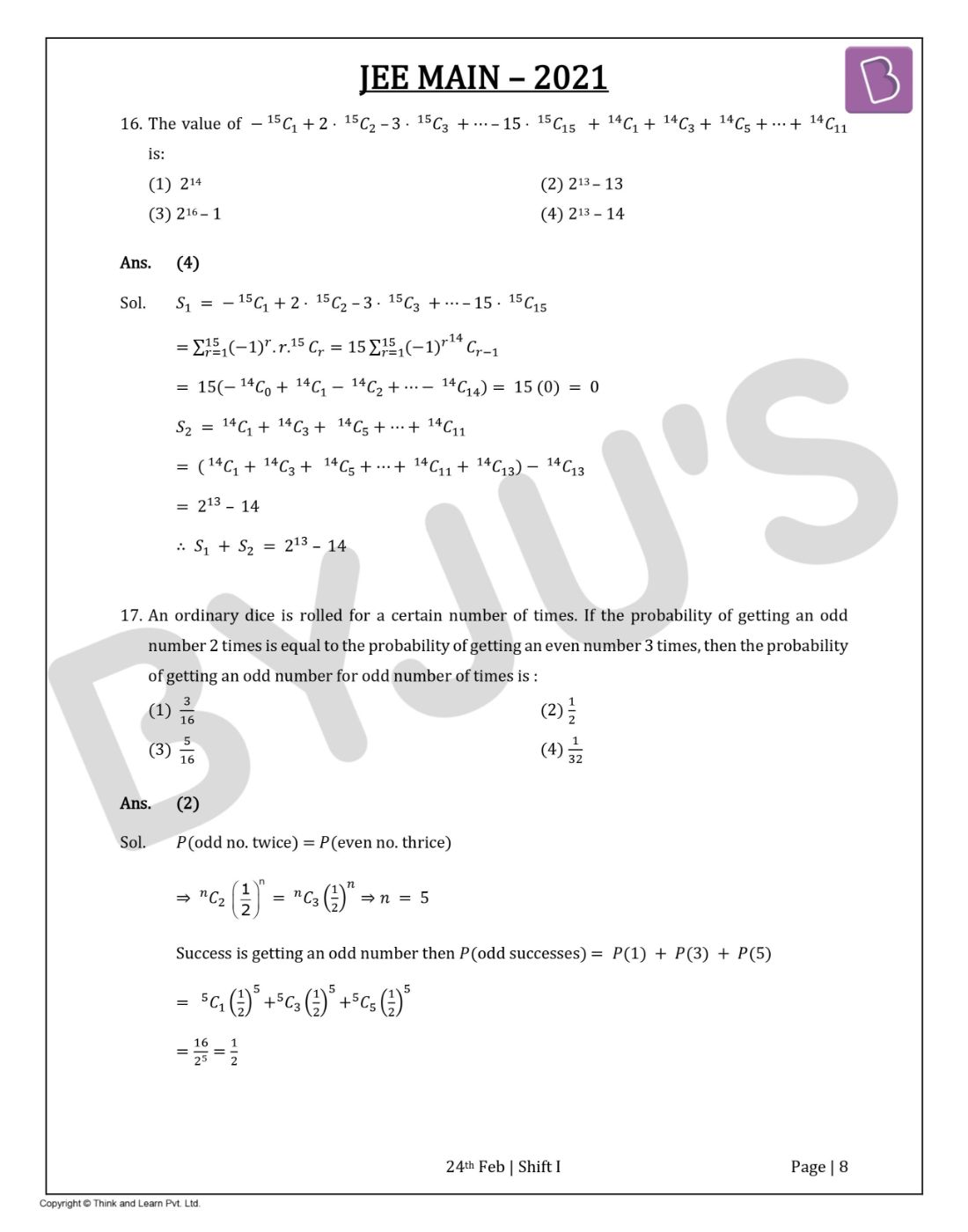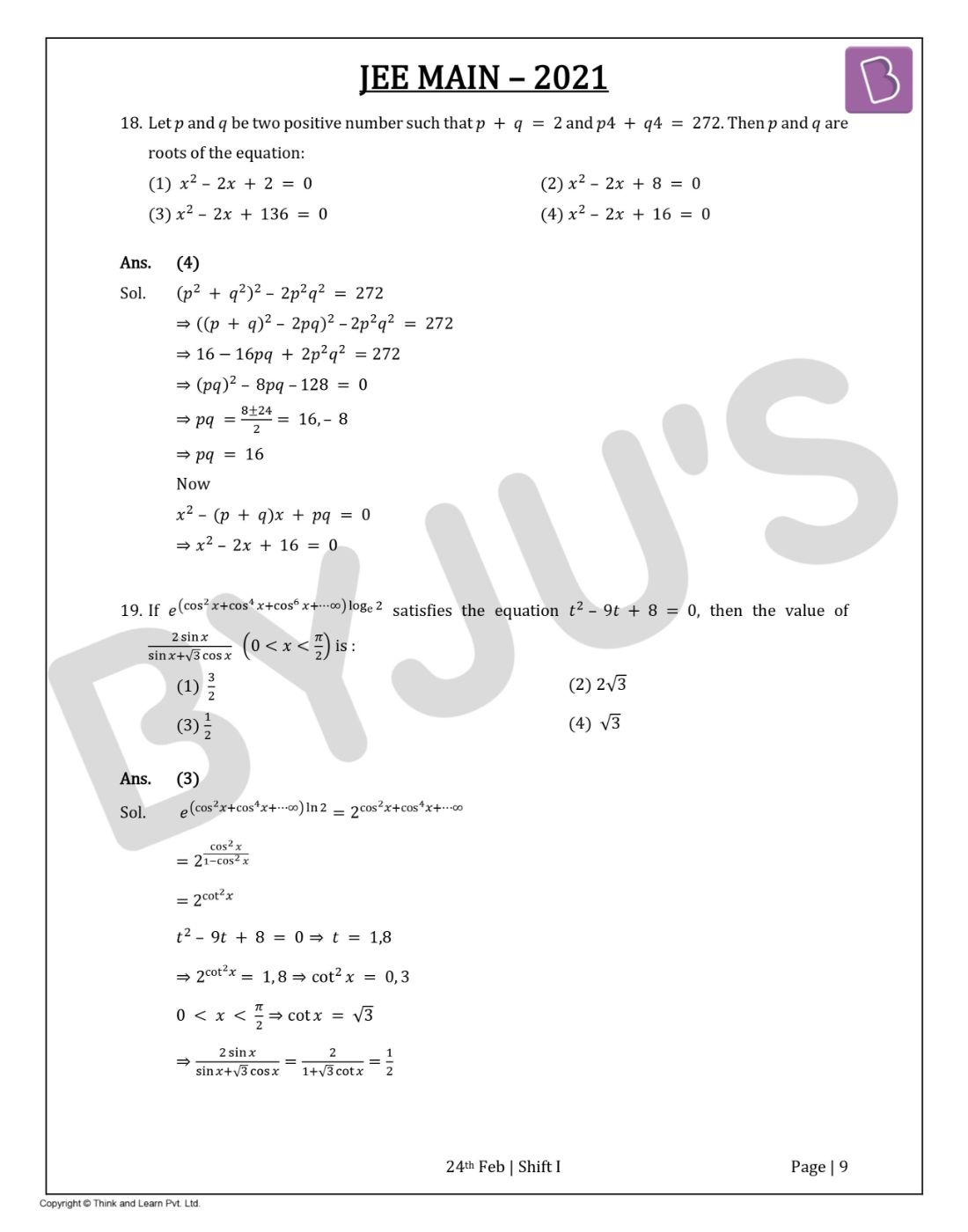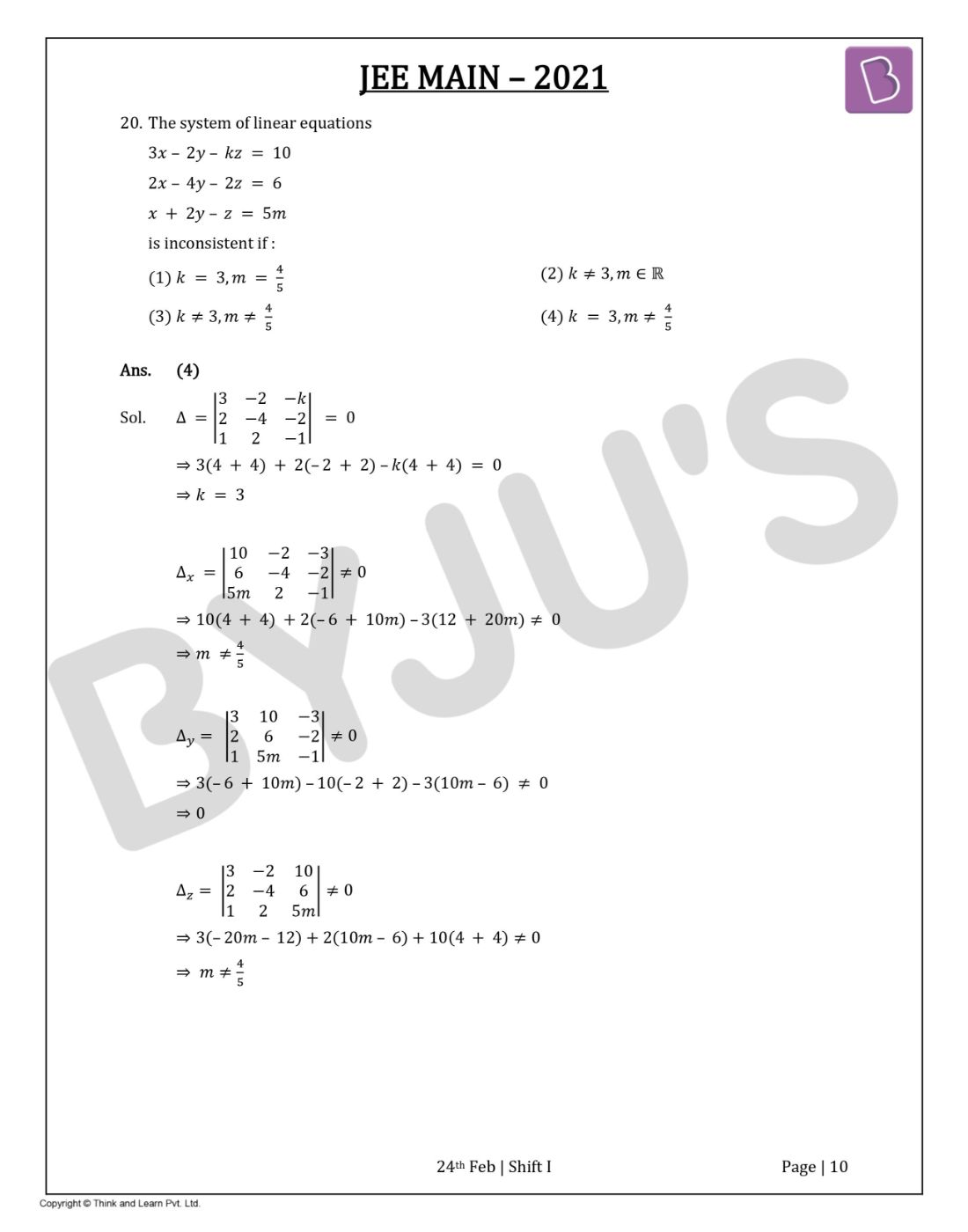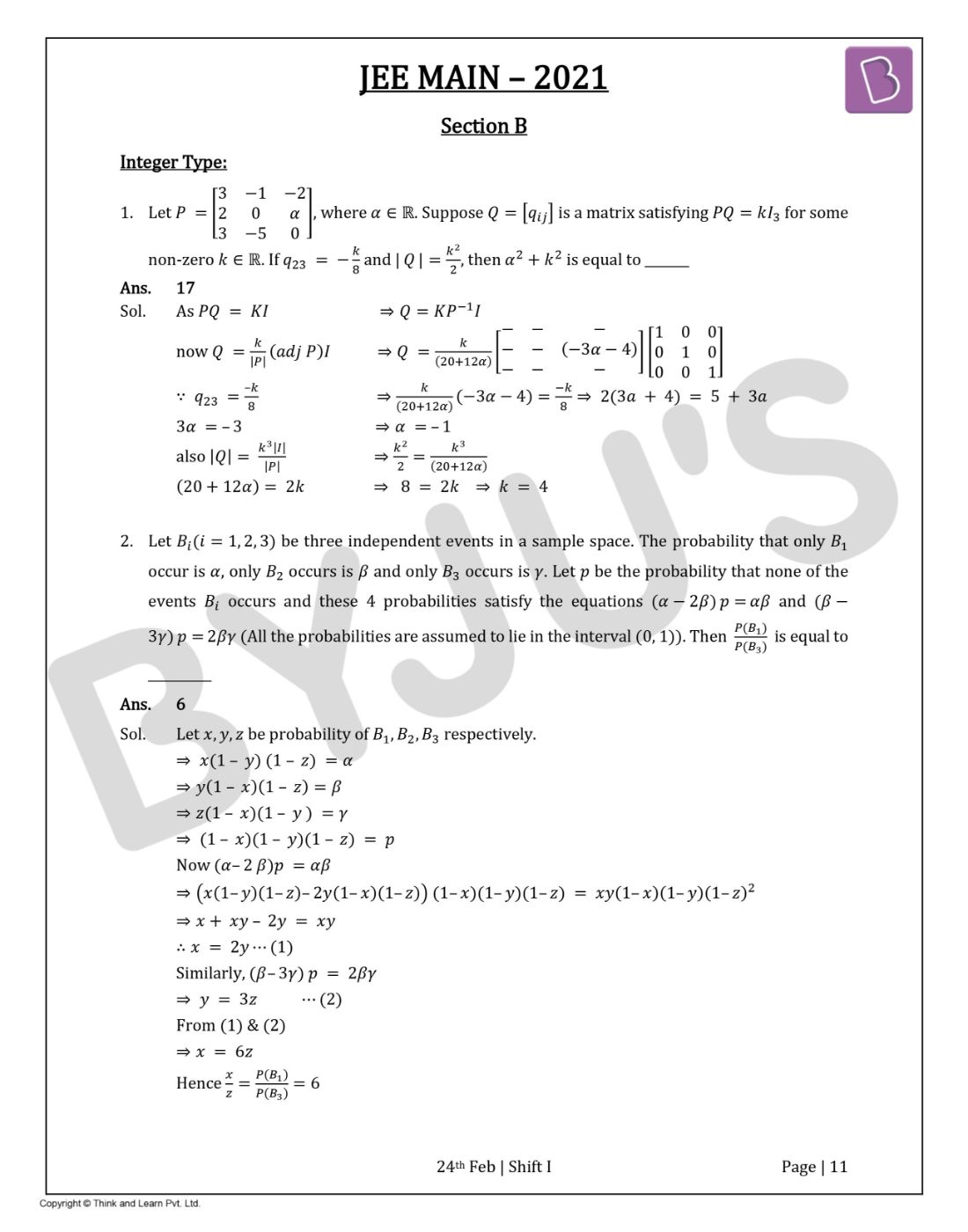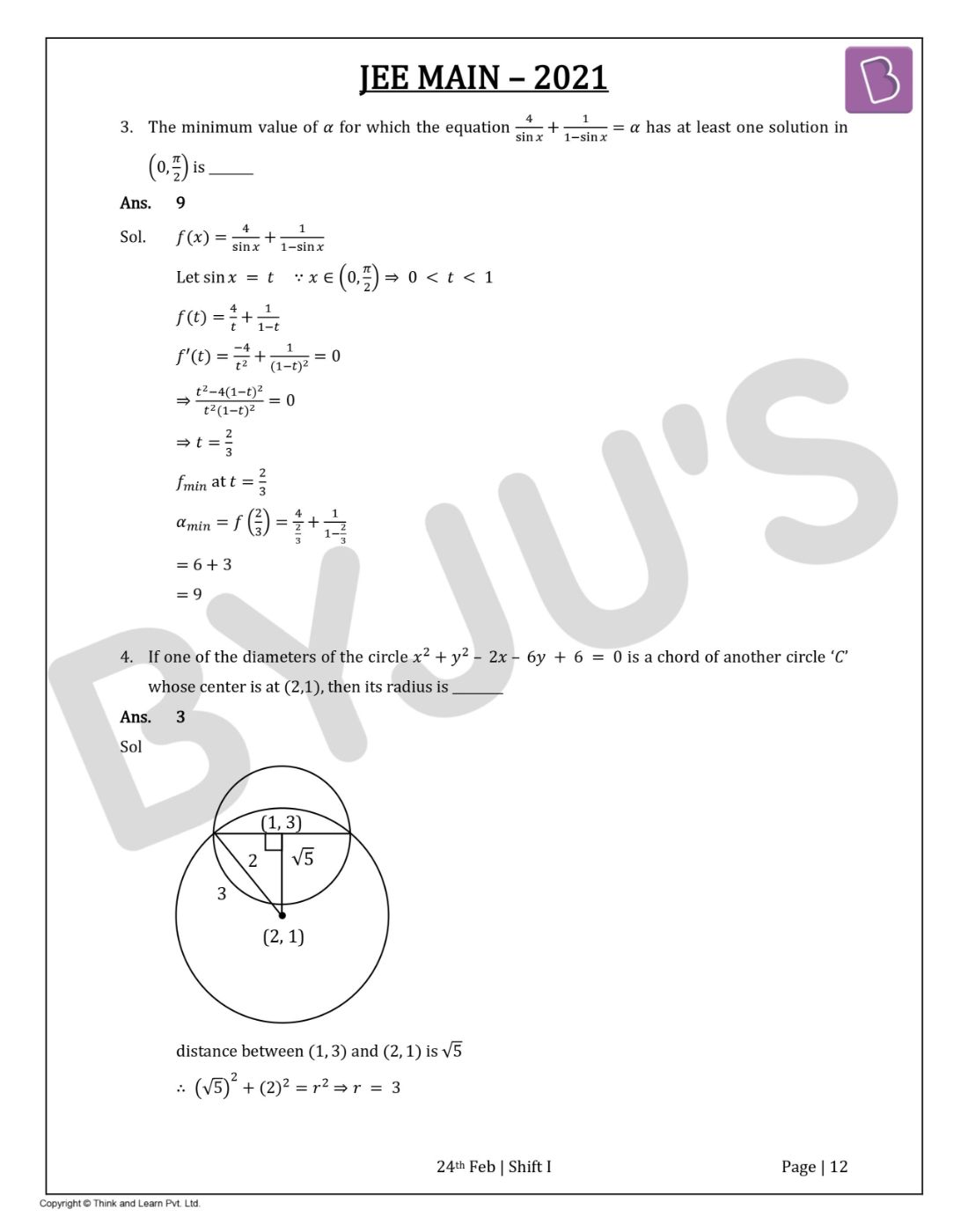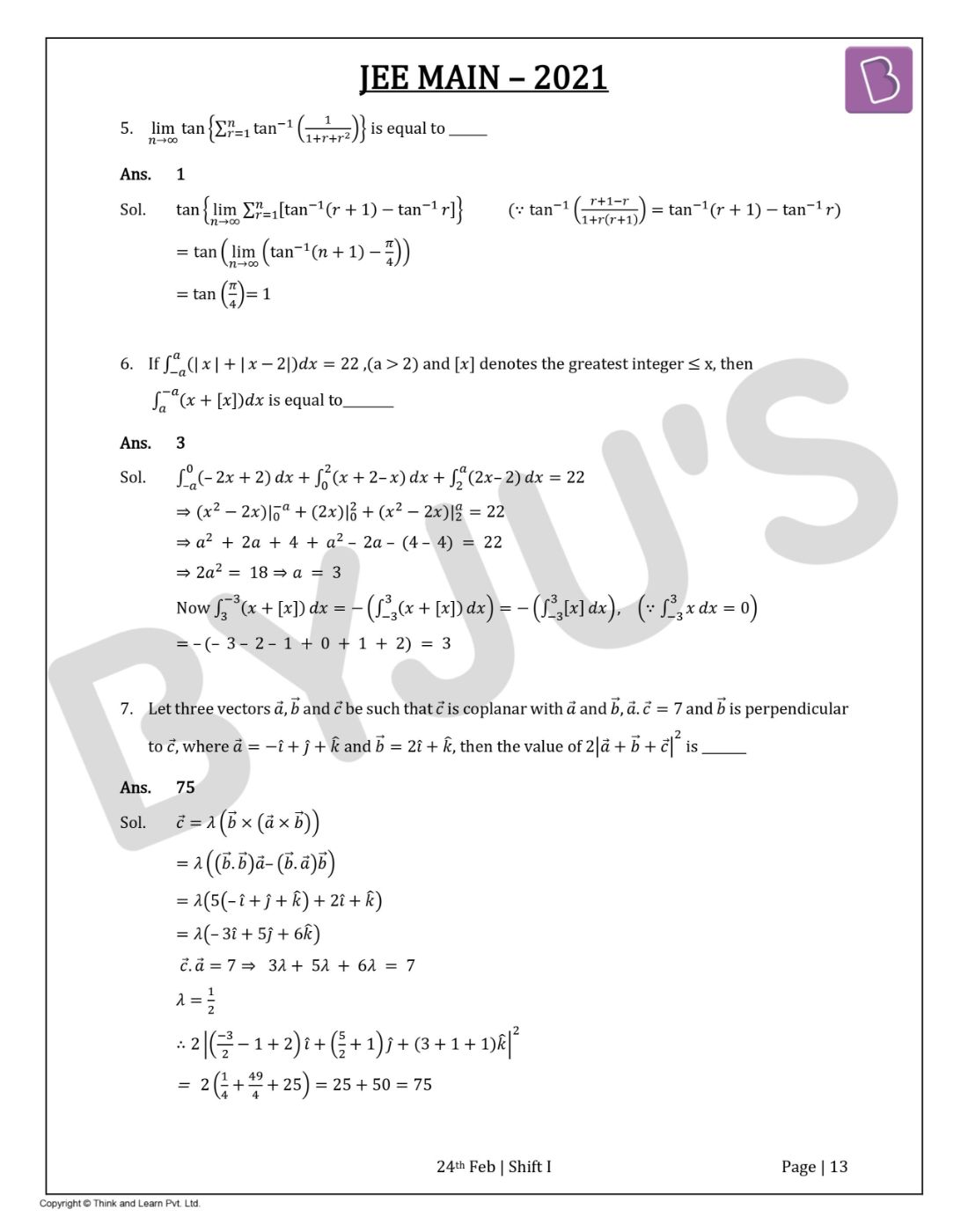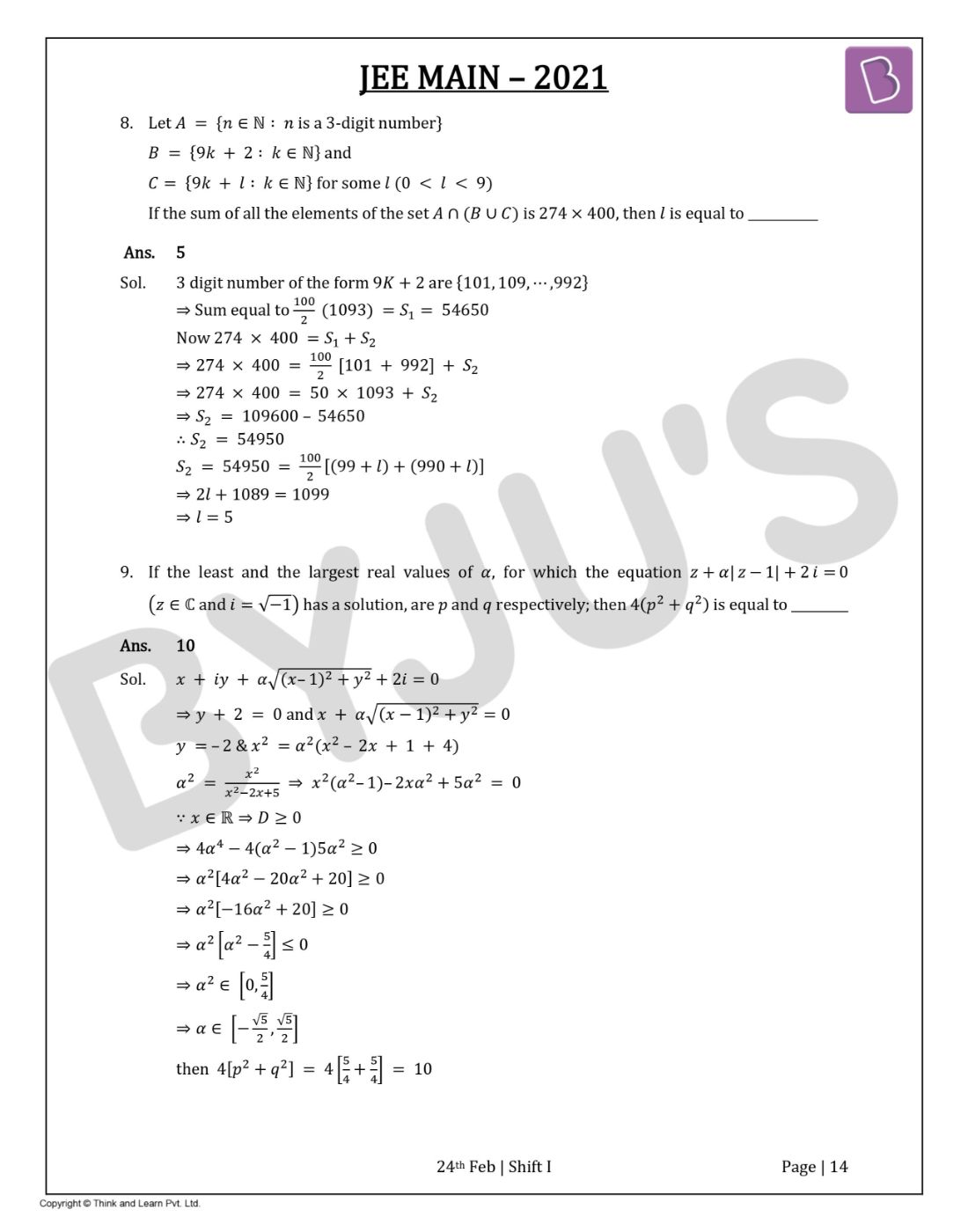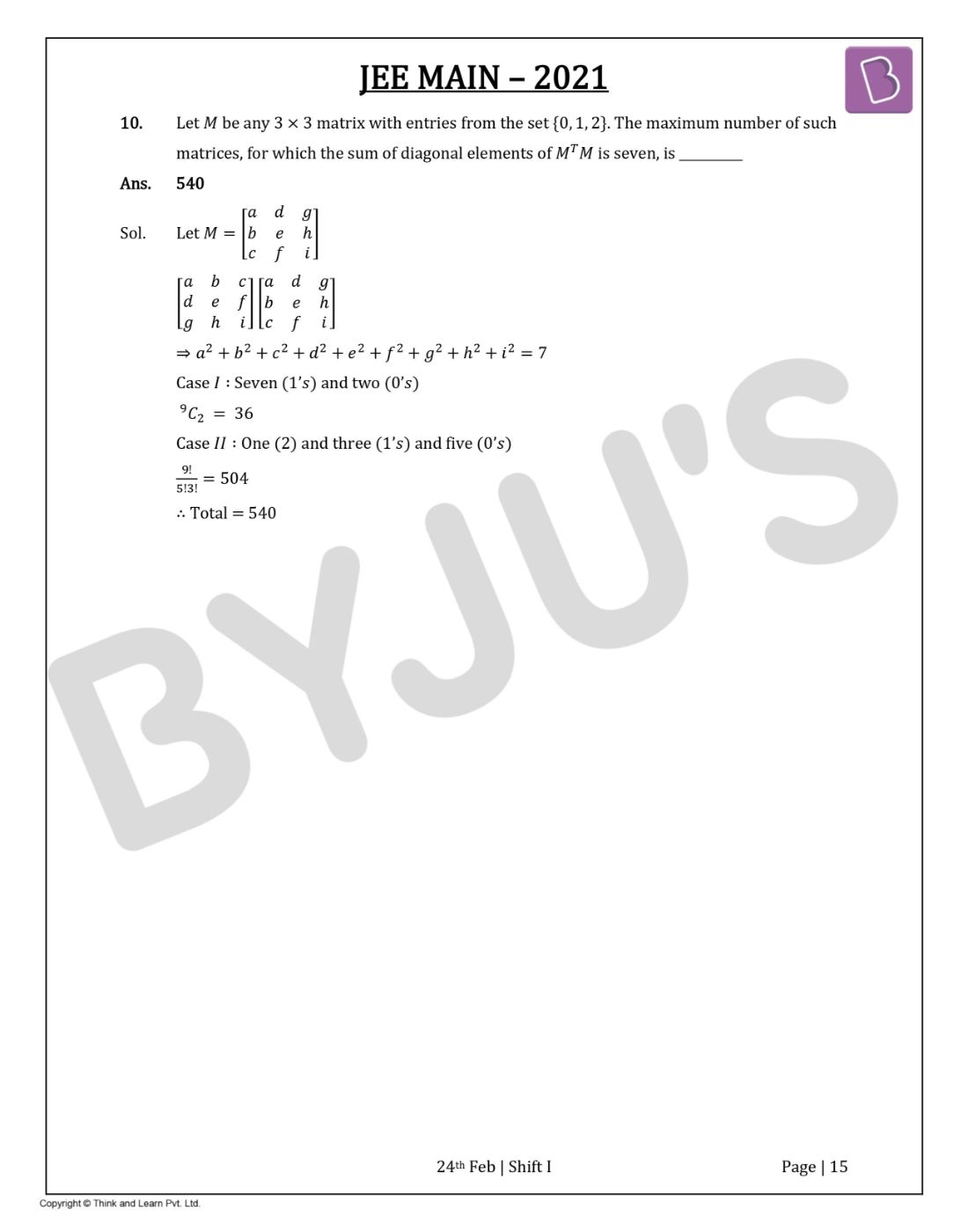Gauge your potential with Aakash BYJU'S JEE Main'23 Mock Test Gauge your potential with Aakash BYJU'S JEE Main'23 Mock Test

# JEE Main 2021 Maths Paper With Solutions Feb 24 Shift 1

Here we have updated the JEE Main 2021 Maths question paper with solutions for Feb 24 session shift 1 on this page. Students can view the latest question paper directly below or download it in the form of a PDF for future use. The questions of JEE Main 2021 Maths question paper have been thoughtfully solved by our top JEE subject experts wherein students will reap the benefit of finding only the most accurate answers.

With such comprehensive preparation from our end and detailed explanations, students will further be able to understand the topics more clearly and they will also learn how to come up with the right solutions for all types of questions that may be asked in the JEE Main Maths question paper. Further with enough practice students will be able to develop better problem solving and time management skills. This is crucial from an examination point of view. Students can go through the question paper and solutions below.

JEE Main 2021 24th February Shift 1 Maths Question Paper

SECTION A

Question 1: The locus of the mid-point of the line segment joining the focus of the parabola y2 = 4ax to a moving point of the parabola, is another parabola whose directrix is:

a. x = a
b. x = 0
c. x = -a/2
d. x = a/2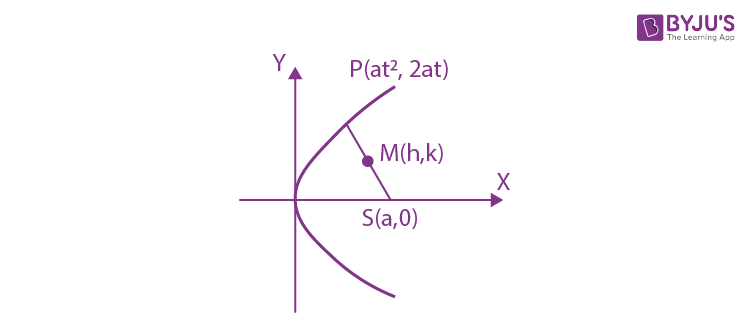h = (at2+a)/2, k = (2at+0)/2

⇒ t2 =(2h-a)/a and t=k/a

⇒ k2/a2 =(2h-a)/a

⇒ Locus of (h, k) is y2 = a (2x – a)

⇒ y2 = 2a(x- a/2)

Its directrix is x – a/2 = -a/2

⇒ x = 0

Question 2: A scientific committee is to formed from 6 Indians and 8 foreigners, which includes at least 2 Indians and double the number of foreigners as Indians. Then the number of ways, the committee can be formed is:

a. 560
b. 1050
c. 1625
d. 575

(2I,4F)+ (3I,6F) + (4I,8F)

=

$$\begin{array}{l}^6C_2\ ^8C_4 + ^6C_3\ ^8C_6 + ^6C_4\ ^8 C_8\end{array}$$

= 15 × 70 + 20 × 28 + 15 × 1

= 1050 + 560 + 15 = 1625

Question 3: The equation of the plane passing through the point (1,2,–3) and perpendicular to the planes 3x + y – 2z = 5 and 2x – 5y – z = 7, is:

a. 3x – 10y – 2z + 11 = 0
b. 6x – 5y – 2z – 2 = 0
c. 11x + y + 17z + 38 = 0
d. 6x – 5y + 2z + 10 = 0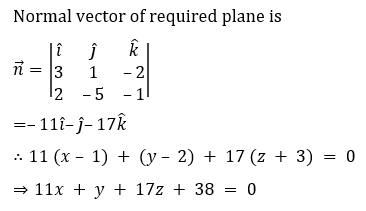Question 4: A man is walking on a straight line. The arithmetic mean of the reciprocals of the intercepts of this line on the coordinate axes is 1/4. Three stones A, B and C are placed at the points (1,1),(2,2), and (4,4) respectively. Then which of these stones is/are on the path of the man?

a. B only
b. A only
c. the three
d. C only

x/a + y/b = 1

h/a + k/b = 1 ⋯⋯(1)

and (1/a + 1/b)/2 = 1/4

∴1/a + 1/b = 1/2 ⋯⋯(2)

(From (1) and (2))

Line passes through fixed point B(2, 2)

Question 5: The statement among the following that is a tautology is:

a. A∧(A∨B)
b. B→[A∧(A→B)]
c. A∨(A∧B)
d. [A∧(A→B)]→B

A∧ (~ A∨B)→B

= [(A∧~A)∨(A∧B)]→ B

= (A∧ B)→ B

= ~ (A∧B)∨B

= t

Question 6: Let f :R→R be defined as f(x) = 2x-1 and g:R – {1} →R be defined as g(x) = (x-½)/(x-1).

Then the composition function f(g(x)) is:

a. Both one-one and onto
b. onto but not one-one
c. Neither one-one nor onto
d. one-one but not onto

f(g(x)) = 2g(x) – 1

= 2(x – 1/2)/(x – 1) – 1

= x/(x – 1)

f(g(x)) = 1 + 1/(x – 1)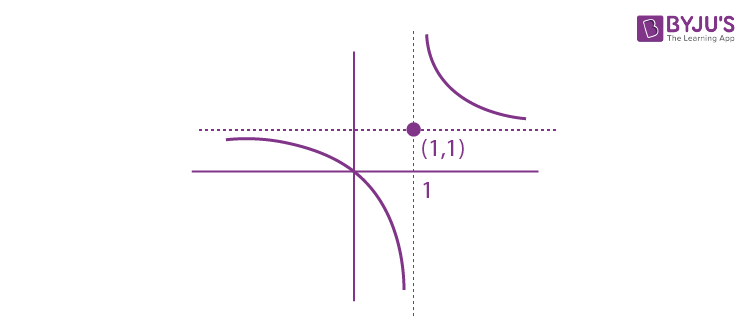∴ one-one, into

Question 7: If f:R→ R is a function defined by f(x) = [x – 1] cos ((2x – 1)/2)𝜋 , where [.] denotes the greatest integer function, then f is:

a. discontinuous only at x = 1
b. discontinuous at all integral values of x except at x = 1
c. continuous only at x = 1
d. continuous for every real x

Doubtful points are x = n, n∈I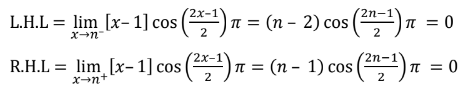f(n) = 0

Hence continuous.

Question 8: The function f(x) = (4x3– 3x2)/6 – 2sin x + (2x – 1)cos x:

a. increases in [1/2, ∞)
b. decreases (-∞, 1/2]
c. increases in (-∞, 1/2]
d. decreases [1/2, ∞)

f’(x) = (2x – 1) (x – sin x )

⇒ f’x ≥ 0 in x∈(-∞, 0] ⋃ [1/2, ∞)

and f’x ≤ 0 in x∈(0, ½)

Question 9: The distance of the point (1, 1, 9) from the point of intersection of the line (x – 3)/1 = (y – 4)/2 = (z – 5)/2 and the plane x + y + z = 17 is:

a. √38
b. 19√2
c. 2√19
d. 38

(x – 3)/1 = (y – 4)/2 = (z – 5)/2 = λ

x = λ + 3, y = 2λ+ 4, z = 2λ+5

Which lies on given plane hence

⇒ λ+3+2λ +4+2λ+5 = 17

⇒ λ = 5/5 = 1

Hence, point of intersection is Q (4, 6, 7)

∴ Required distance =PQ

= √(9+25+4)

= √38

Question 10: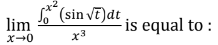a. 2/3
b. 0
c. 1/15
d. 3/2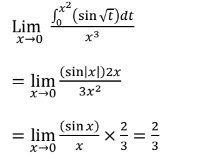Question 11: Two vertical poles are 150 m apart and the height of one is three times that of the other. If from the middle point of the line joining their feet, an observer finds the angles of elevation of their tops to be complementary, then the height of the shorter pole (in meters) is:

a. 25
b. 20√3
c. 30
d. 25√3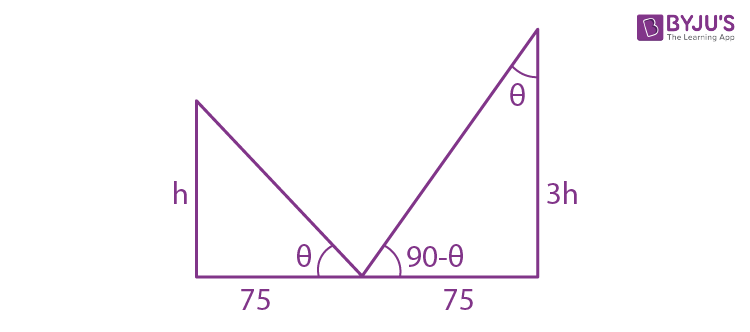tan θ = h/75 = 75/3h

h2 = 752/3

h = 25√3m

Question 12: If the tangent to the curve y = x3 at the point P(t, t3) meets the curve again at Q, then the ordinate of the point which divides PQ internally in the ratio 1:2 is:

a. –2t3
b. –t3
c. 0
d. 2t3

Equation of tangent at P(t, t3)

(y – t3) = 3t2(x – t) ⋯(1)

Now solve the above equation with

y = x3 ⋯(2)

By (1) & (2)

x3 – t3 = 3t2 (x – t)

x2 + xt + t2 = 3t2

x2 + xt – 2t2 = 0

⇒(x – t)(x + 2t) = 0

⇒x = – 2t

⇒Q(-2t, -8t3)

Ordinate of required point = (2t3 + (-8t)3)/3

= -2t3

Question 13: The area (in sq.units) of the part of the circle x2 + y2 = 36, which is outside the parabola y2 = 9x, is:

a. 24π + 3√3
b. 12π + 3√3
c. 12π – 3√3
d. 24π – 3√3

The curves intersect at point (3, ± 3√3)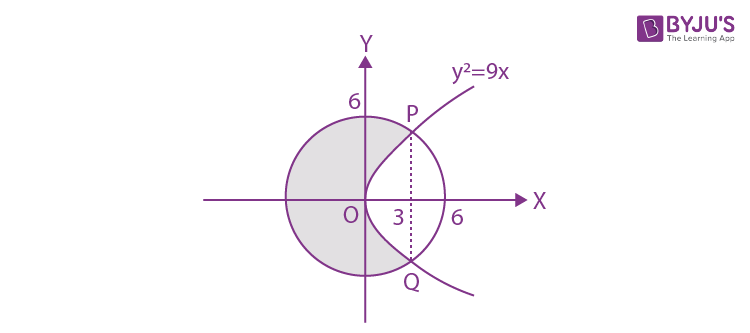Required area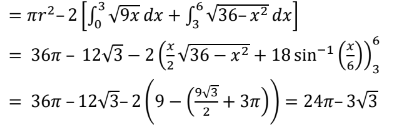Question 14: If ∫(cos x -sin x)/√(8-sin 2x) dx = a sin-1(sin x + cos x)/b + c where c is a constant of integration, then the ordered pair (a, b) is equal to:

a. (1, –3)
b. (1, 3)
c. (–1, 3)
d. (3, 1)

Put sin x + cos x = t ⇒1 + sin 2x = t2

(cos x -sin x ) dx = dt

⇒I = ∫dt/√(8-(t2-1))

= ∫dt/(9-t2)

= sin-1 (t/3) + c = sin-1(sin x + cos x)/3 + c

⇒ a = 1 and b = 3

Question 15: The population P = P(t) at time ‘t’ of a certain species follows the differential equation dP/dt = 0.5P – 450. If P(0) = 850, then the time at which population becomes zero is:

a. ½ loge 18
b. 2loge 18
c. loge 9
d. loge 18

dp/dt = (p-900)/2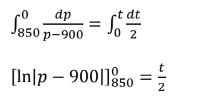ln |900| – ln |50| = t/2

t/2 = ln |18|

⇒ t = 2ln 18

Question 16: The value of – 15C1 + 2. 15C2 – 3.15C3+ ….-15.15C1 + 14C1+ 14C3 + 14C5 + …14C11 is

a. 214
b. 213 – 13
c. 216 – 1
d. 213 – 14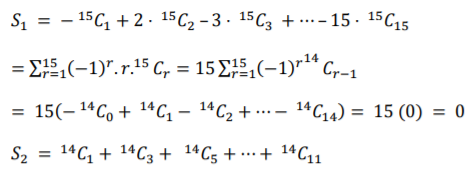= (14C1+ 14C3 + 14C5 + …14C11 + 14C13) – 14C13

= 213 – 14

∴ S1 + S2 = 213 – 14

Question 17: An ordinary dice is rolled for a certain number of times. If the probability of getting an odd number 2 times is equal to the probability of getting an even number 3 times, then the probability of getting an odd number for odd number of times is:

a. 3/16
b. 1/2
c. 5/16
d. 1/32

P(odd no. twice) = P(even no. thrice)

nC2 (1/2)n = nC3 (1/2)n

⇒ n = 5

Success is getting an odd number then P(odd successes) = P(1) + P(3) + P(5)

= 5C1(1/2)5 + 5C3(1/2)5+ 5C5(1/2)5

= 16/25

= 1/2

Question 18: Let p and q be two positive number such that p + q = 2 and p4 + q4 = 272. Then p and q are roots of the equation:

a. x2 – 2x + 2 = 0
b. x2 – 2x + 8 = 0
c. x2 – 2x + 136 = 0
d. x2 – 2x + 16 = 0

(p2 + q2)2 – 2p2q2 = 272

((p + q)2 – 2pq)2 – 2p2q2 = 272

⇒16 – 16pq + 2p2q2 = 272

(pq)2 – 8pq –128 = 0

⇒pq = (8±24)/2 = 16, – 8

⇒pq = 16

Now

x2 – (p + q)x + pq = 0

x2 – 2x + 16 = 0

Question 19: If

$$\begin{array}{l}e^{(cos^{2}x + cos^{4}x + cos^{6}x + …..\infty )log_{e}2}\end{array}$$
satisfies the equation t2 – 9t + 8 = 0, then the value of 2sin x/(sin x + √3cos x) 0< x<π/2 is:

a. 3/2
b. 2√3
c. 1/2
d. √3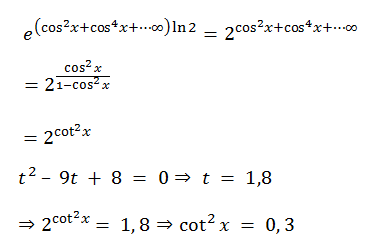0 < x < π/2 ⇒ cot⁡x = √3

⇒(2 sin⁡x)/(sin⁡x+√3 cos⁡x )

= 2/(1+√3 cot ⁡x)

= 1/2

Question 20: The system of linear equations

3x – 2y – kz = 10

2x – 4y – 2z = 6

x + 2y – z = 5m

is inconsistent if :

a. k = 3, m = 4/5
b. k ≠ 3,m∈R
c. k ≠ 3, m ≠ 4/5
d. k = 3, m ≠ 4/5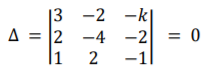⇒ 3(4 + 4) + 2(–2 + 2) -k(4 + 4) = 0

⇒ k = 3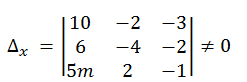⇒ 10(4 + 4) +2(-6 + 10m) -3(12 + 20m) ≠ 0

⇒ m ≠ 4/5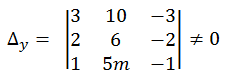⇒ 3(-6 + 10m) – 10(- 2 + 2) – 3(10m – 6) ≠ 0

⇒ 0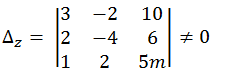⇒3(-20m – 12) +2(10m – 6) +10(4 + 4) ≠ 0

⇒ m ≠ 4/5

Section B

Question 1: Let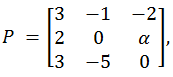where α∈R. Suppose Q = [qij ] is a matrix satisfying PQ = kI3 for some non-zero k∈R. If q23= – k/8 and |Q⁡| = k2/2, then α2 + k2 is equal to ___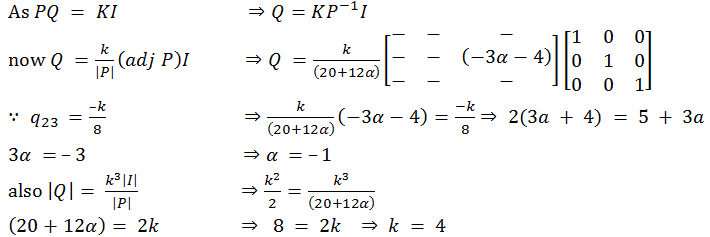Question 2: Let Bi (i = 1,2,3) be three independent events in a sample space. The probability that only B1 occur is α, only B2 occurs is β and only B3 occurs is γ. Let p be the probability that none of the events Bi occurs and these 4 probabilities satisfy the equations (α – 2β)p ⁡= αβ and (β – 3γ)p⁡= 2βγ (All the probabilities are assumed to lie in the interval (0, 1)). Then (P(B1⁡))/(P(B3⁡) )is equal to _____

Let x,y,z be probability of B1, B2, B3 respectively.

⇒ x(1 – y) (1 – z) = α

⇒ y(1 – x)(1 – z) = β

⇒ z(1 – x)(1 – y ) = γ

⇒ (1 – x)(1 – y)(1 – z) = p

Now (α – 2β)p = αβ

⇒ (x(1–y)(1–z)-2y(1-x)(1–z)) (1–x)(1–y)(1–z) = xy(1–x)(1–y) (1–z)2

⇒ x+ xy – 2y = xy

∴x = 2y⋯(1)

Similarly, (β–3γ) p = 2βγ

⇒ y = 3z ⋯(2)

From (1) & (2)

⇒x = 6z

Hence x/z = P(B1)/P(B3) = 6

Question 3: The minimum value of α for which the equation 4/sin⁡x +1/(1-sin⁡x )=α has at least one solution in (0,π/2) is _______

f(x⁡) = 4/sin⁡x + 1/(1 – sin⁡x )

Let sin⁡x = t ∵x∈(0, π/2) ⇒ 0 < t < 1

f(t⁡)= 4/t + 1/(1-t)

f'(t⁡) = (-4)/t2 + 1/(1 – t⁡)2 = 0

⇒(t2-4(1-t⁡)2/t2(1-t⁡)2 = 0

⇒t = 2/3

fmin at t = 2/3

αmin = f(2/3) =4/(2/3) + 1/(1 – 2/3)

= 6 + 3

= 9

Question 4: If one of the diameters of the circle x2+y2 – 2x – 6y + 6 = 0 is a chord of another circle ‘C’ whose center is at (2,1), then its radius is ________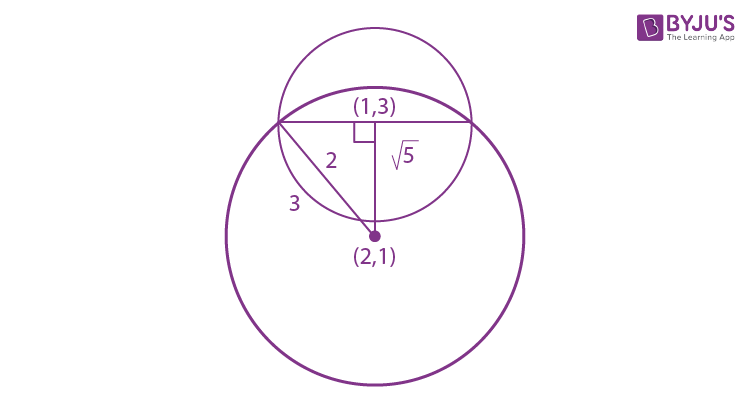distance between (1,3) and (2,1) is √5

∴ (√5)2+(2)2= r2

⇒r = 3

Question 5: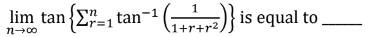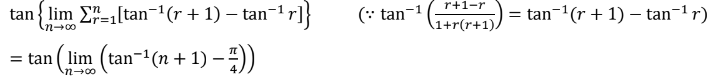= tan 𝜋/4

= 1

Question 6: If

$$\begin{array}{l}\int_{-a}^{a}(\left | x \right |+\left | x-2 \right |)dx = 22\end{array}$$
and [x] denotes the greatest integer ≤ x, then
$$\begin{array}{l}\int_{a}^{-a}(x+\left [ x \right ])dx\end{array}$$
is equal to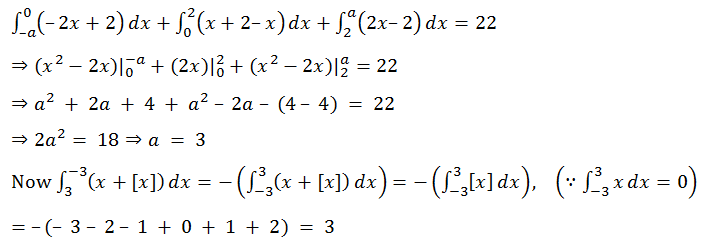Question 7: Let three vectors

$$\begin{array}{l}\vec{a}\end{array}$$
,
$$\begin{array}{l}\vec{b}\end{array}$$
and
$$\begin{array}{l}\vec{c}\end{array}$$
be such that
$$\begin{array}{l}\vec{c}\end{array}$$
is coplanar with
$$\begin{array}{l}\vec{a}\end{array}$$
and
$$\begin{array}{l}\vec{b}\end{array}$$
,
$$\begin{array}{l}\vec{a}.\vec{c}=7\end{array}$$
and
$$\begin{array}{l}\vec{b}\end{array}$$
is perpendicular to
$$\begin{array}{l}\vec{c}\end{array}$$
, where
$$\begin{array}{l}\vec{a} = -\hat{i}+\hat{j}+\hat{k}\end{array}$$
and
$$\begin{array}{l}\vec{b} = 2\hat{i}+\hat{k}\end{array}$$
, then the value of
$$\begin{array}{l}2\left | \vec{a} +\vec{b}+\vec{c} \right |^{2}\end{array}$$
is _______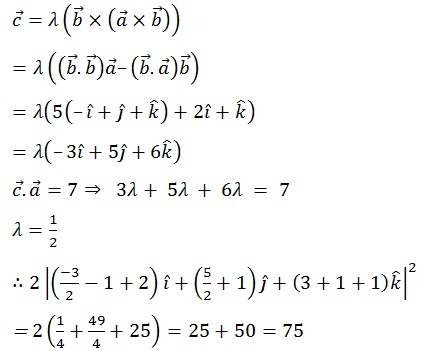Question 8: Let A = {n∈N∶ n is a 3-digit number}, B = {9k + 2∶ k∈N} and C = {9k + l∶ k∈N} for some l(0 < l < 9). If the sum of all the elements of the set A∩(B∪C) is 274×400, then l is equal to ___________

3 digit number of the form 9K+2 are {101,109,⋯,992}

⇒ Sum equal to (100/2)(1093) = S1= 54650

Now 274 × 400 =S1+S2

⇒274 × 400 = (100/2) [101 + 992] + S2

⇒274 × 400 = 50 × 1093 + S2

⇒S2 = 109600 – 54650

∴S2 = 54950

S2 = 54950 = (100/2) [(99+l) + (990+l)]

⇒ 2l + 1089 = 1099

⇒ l = 5

Question 9: If the least and the largest real values of α, for which the equation z + α|z⁡-1| + 2 i⁡ = 0 (z∈C and i =√(-1)) has a solution, are p and q respectively; then 4(p2 + q2) is equal to _________

x + iy + α√((x–1)2 +y2) + 2i=0

⇒y + 2 = 0 and x + α√((x-1)2+y2)=0

y = –2 & x2 = α2(x2 – 2x + 1 + 4)

α2 = x2/(x2– 2x + 5)

⇒ x22 – 1) – 2xα2 + 5α2 = 0

∵x∈R ⇒ D≥0

⇒ 4α4 – 4(α2 – 1)5α2 ≥ 0

⇒ α2 [4α2 – 20α2 + 20] ≥ 0

⇒ α2 [-16α2 + 20] ≥ 0

⇒ α22 – 5/4] ≤ 0

⇒ α2 ∈ [0, 5/4]

⇒ α ∈ [-√5/2, √5/2]

then 4[p2 + q2] = 4[5/4 + 5/4]

= 10

Question 10: Let M be any 3×3 matrix with entries from the set {0,1,2}. The maximum number of such matrices, for which the sum of diagonal elements of MTM is seven, is __________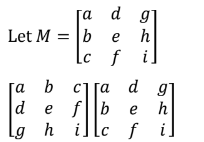⇒ a2 + b2 + c2 + d2 + e2 + f2 + g2 + h2 + i2 = 7

Case I∶ Seven (1’s) and two (0’s)

9C2 = 36

Case II∶ One (2) and three (1’s) and five (0’s)

9!/5!3! = 504

∴Total = 540